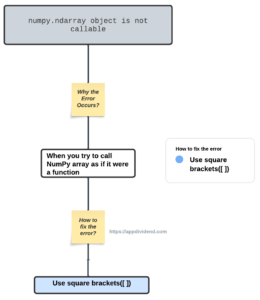# How to Fix TypeError: ‘numpy.ndarray’ object is not callableDiagram

To fix the TypeError: ‘numpy.ndarray’ object is not callable error, you can use the “square brackets([ ])” to access array elements in the numpy array and avoid using the round(( )) brackets.

Python raises the TypeError: ‘numpy.ndarray’ object is not callable error when you “try to call a NumPy array as if it were a function”. This occurs if you use round brackets ( ) instead of square brackets [ ] to retrieve items from a list.

``````import numpy as np

arr = np.array([11, 21, 19, 46])

print(arr())``````

Output

``TypeError: 'numpy.ndarray' object is not callable``

Using the np.array() method, we created an array and used that array as a function, and called it using the double parenthesis.

The ndarrays are not functions, so you can’t call it the way you call a normal function.

## How to fix TypeError: ‘numpy.ndarray’ object is not callable

``````import numpy as np

arr = np.array([11, 21, 19, 46])

print(arr)``````

Output

``19``

In this code, we access the third element of the array, which is 19. Array indexing starts with 0, so the third element will have a “2” index. And that’s how you resolve this kind of TypeError.

Numpy ndarrays can store and manipulate large arrays of homogeneous data in Python.

Python does not have arrays, but using a third-party library like Numpy can use this type of data structure in our application.

### Alternate solution

Use the appropriate method or attribute of the ndarray object to access or manipulate array data.

For example, ndim, size, and shape are methods you can apply on a ndarray object as it is.

``````import numpy as np

arr = np.array([11, 21, 19, 46])

print(arr.size)``````

Output

``4``

The array has four elements, and its size is 4. That’s why the size attribute returns 4 as output.

That’s it.